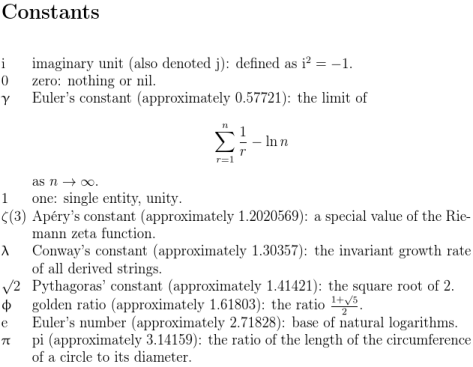# Gallery: ConstantsThis example is taken from the “Examples” chapter of bib2gls’s user manual.

The entries are defined in a .bib file, constants.bib. Instead of using the standard entry types (such as @entry) and field names (such as name) recognised by bib2gls, these entries are all defined using a custom field @constant and custom field names (constantname, constantsymbol, definition, alternative, identifier and value). These can be aliased on a per-document basis to allow for greater flexibility. For example, one document might alias the unknown constantname field to the known name field, but another document might alias the unknown constantsymbol to the name field instead.

Some semantic commands are provided in the @preamble:

@preamble{"\providecommand{\constanti}{\mathrm{i}}
\providecommand{\constantj}{\mathrm{j}}
\providecommand{\constante}{\mathrm{e}}
\providecommand{\constantpi}{\uppi}
\providecommand{\constantgamma}{\upgamma}
\providecommand{\constantphi}{\upphi}
\providecommand{\constantlambda}{\uplambda}"}


By placing them within the @preamble of the .bib file, the definitions can be accessed by bib2gls if it needs to interpret them.

The actual entry definitions look like:

@constant{pi,
constantname={pi},
constantsymbol={\ensuremath{\constantpi}},
definition={the ratio of the length of the circumference
of a circle to its diameter},
value={3.14159},
identifier={constant}
}


Some of the entries don’t have the value field set. For example:

@constant{one,
constantname={one},
constantsymbol={\ensuremath{1}},
definition={single entity, unity},
identifier={constant}
}

@constant{imaginary,
constantname={imaginary unit},
constantsymbol={\ensuremath{\constanti}},
definition={defined as $\constanti^2 = -1$},
identifier={constant},
alternative={\ensuremath{\constantj}}
}


Some of these entries use commands from the upgreek package, so a document that uses this .bib file must load that package to ensure they are defined.

This example document aliases @constant to @number with entry-type-aliases={constant=entry} and aliases the unknown fields with field-aliases. The numeric value is aliased to the user1 field, so it’s possible to sort the entries numerically by selecting that field as the sort value.

\GlsXtrLoadResources[
src={constants},% data in constants.bib
% make @constant behave like @number:
entry-type-aliases={constant=number},
field-aliases={
identifier=category,
constantsymbol=name,
constantname=description,
value=user1,
definition=user2,
alternative=user3
},
type=main,
set-widest,
sort-field=user1,
missing-sort-fallback=name,
sort=double,
selection=all
]


(The numeric comparator will treat an empty value as 0).

The post-description category hook, provided by the glossaries-extra package, is used to append an alternative symbol and the approximate value after the description:

\newcommand{\glsxtrpostdescconstant}{%
\glsxtrifhasfield{useriii}{\glscurrententrylabel}%
{ (also denoted \glscurrentfieldvalue
\glsxtrifhasfield{useri}{\glscurrententrylabel}%
{, approximately \glscurrentfieldvalue}%
{}%
)%
}%
{%
\glsxtrifhasfield{useri}{\glscurrententrylabel}%
{ (approximately \glscurrentfieldvalue)}%
{}%
}%
\glsxtrifhasfield{userii}{\glscurrententrylabel}%
{: \glscurrentfieldvalue}%
{}%
}


The complete document code follows:

\documentclass[12pt,a4paper]{article}

\usepackage[T1]{fontenc}
\usepackage{upgreek}

\usepackage[record,% use bib2gls
% load glossary-tree.sty and patch styles:
stylemods={tree},
style=alttree]{glossaries-extra}

src={constants},% data in constants.bib
% make @constant behave like @number
entry-type-aliases={constant=number},
field-aliases={
identifier=category,
constantsymbol=name,
constantname=description,
value=user1,
definition=user2,
alternative=user3
},
type=main,
set-widest,
sort-field=user1,
missing-sort-fallback=name,
sort=double,
selection=all
]

\newcommand{\glsxtrpostdescconstant}{%
\glsxtrifhasfield{useriii}{\glscurrententrylabel}%
{ (also denoted \glscurrentfieldvalue
\glsxtrifhasfield{useri}{\glscurrententrylabel}%
{, approximately \glscurrentfieldvalue}%
{}%
)%
}%
{%
\glsxtrifhasfield{useri}{\glscurrententrylabel}%
{ (approximately \glscurrentfieldvalue)}%
{}%
}%
\glsxtrifhasfield{userii}{\glscurrententrylabel}%
{: \glscurrentfieldvalue}%
{}%
}

\begin{document}
\printunsrtglossary[title={Constants}]
\end{document}

To build the document, you need to run the following commands:
pdflatex bib2gls-constants
bib2gls bib2gls-constants
pdflatex bib2gls-constants


(See Incorporating makeglossaries or makeglossaries-lite or bib2gls into the document build.) Note that bib2gls is not invoked with the --group switch.